# 求解二维Euler方程的旋转通量混合格式*

(长安大学 理学院, 西安 710064)

## 引 言

HLL格式是直接近似单元交界面的数值通量，因而此数值通量是非常鲁棒和有效的Godunov方法．熵稳定格式满足热力学第二定律，可以有效避免伪振荡．因而本文在此基础上提出利用旋转不变性将通量分解为两个正交的方向，每个方向上采用熵稳定格式和HLL格式耦合得到混合的旋转通量格式，最终通过数值算例验证该方法的间断捕捉能力和分辨率.

## 1 控 制 方 程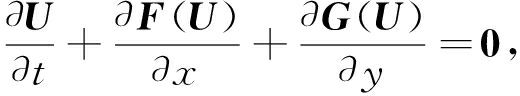(1)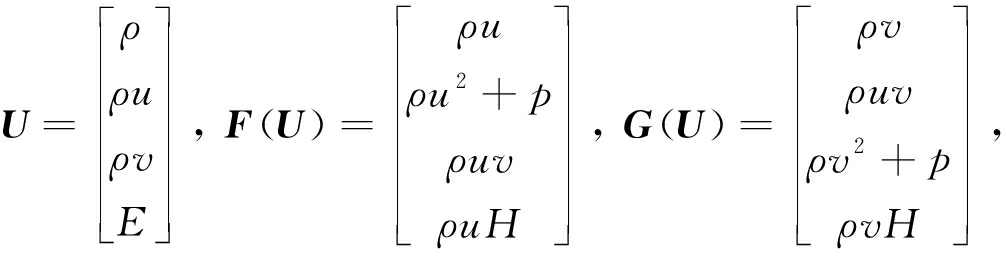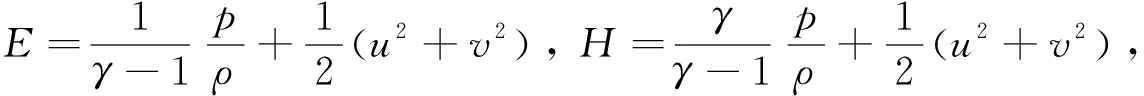## 2 方程的离散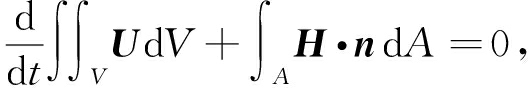(2)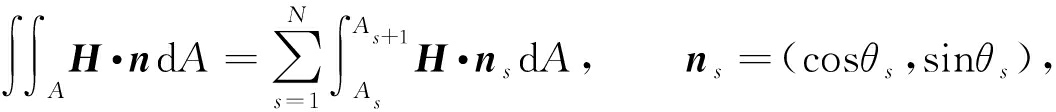(3)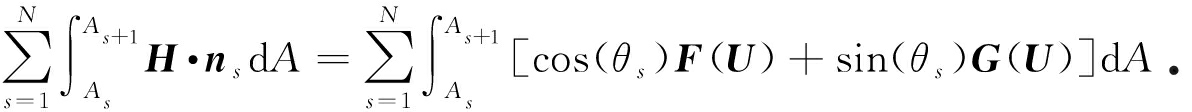(4)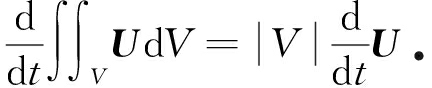(5)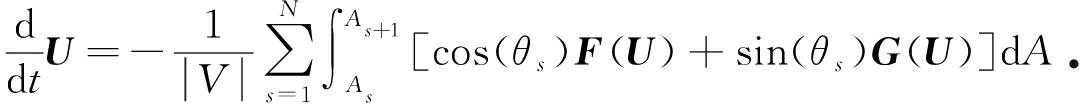(6)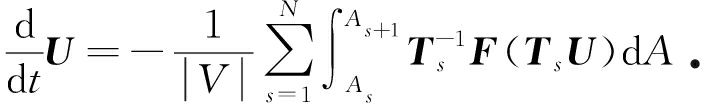(7)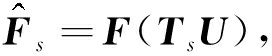则式(7)右端积分的每一项可写为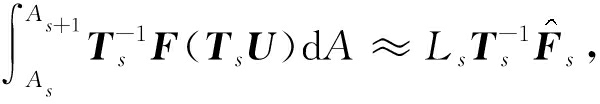LsAsAs+1的长度．则方程(1)的半离散格式为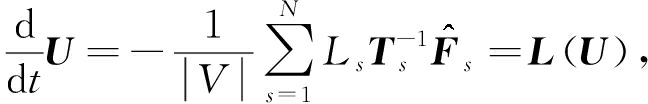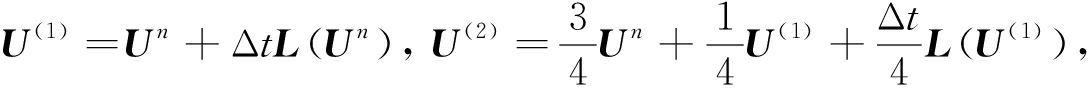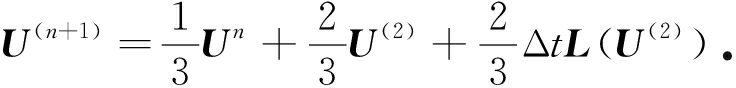## 3 数值通量格式

### 3.1 熵稳定格式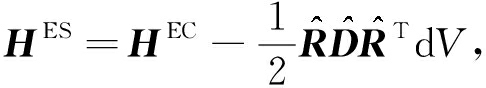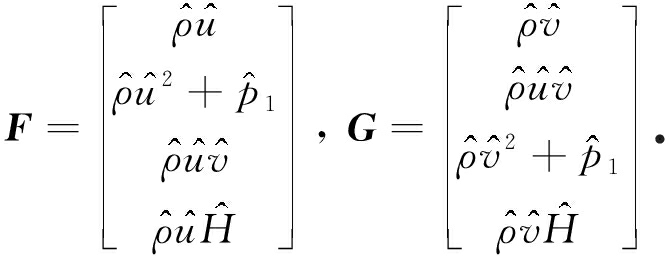Roe根据Euler方程自身的性质，提出了一种结构简单、计算效率高的熵守恒通量函数，该通量函数需选取参变量Z,即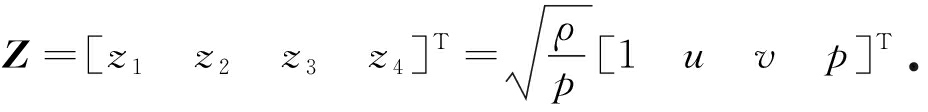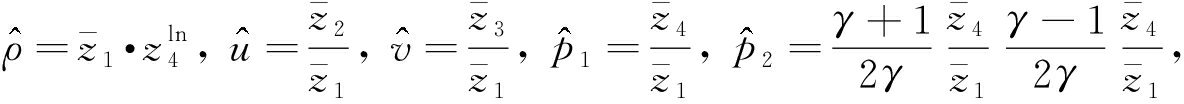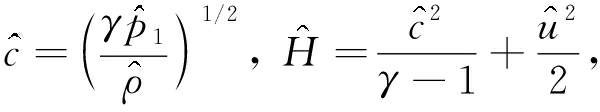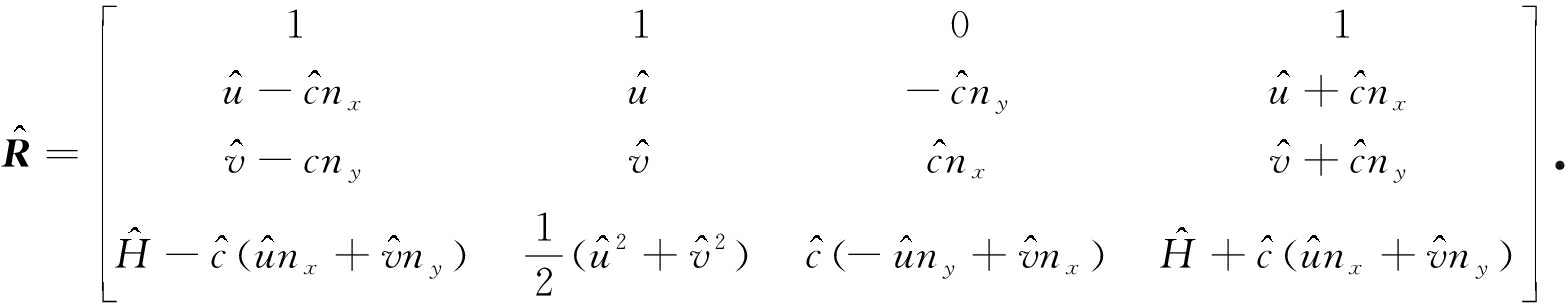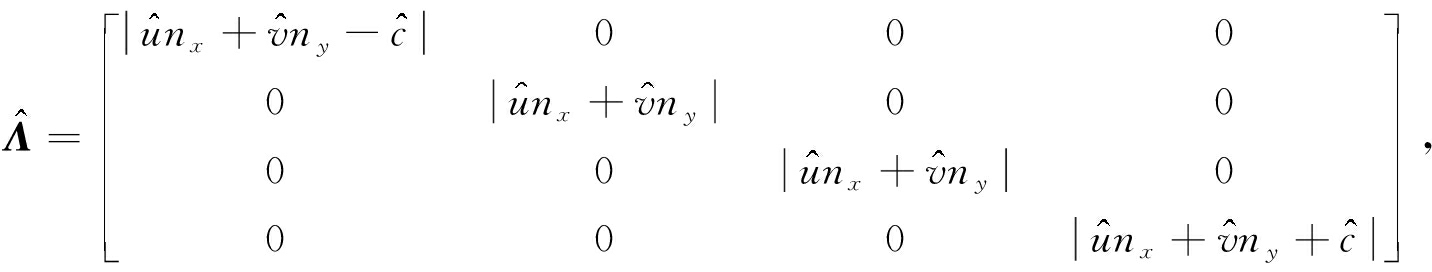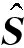是比例系数矩阵，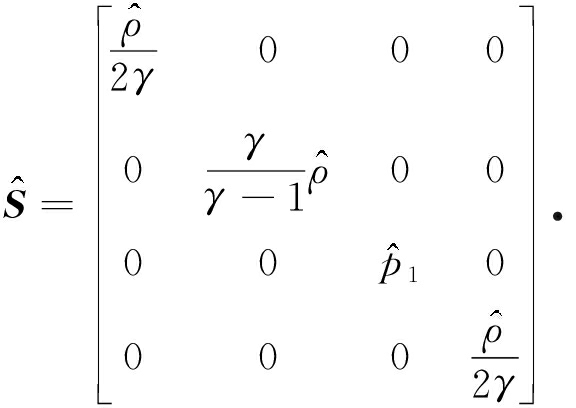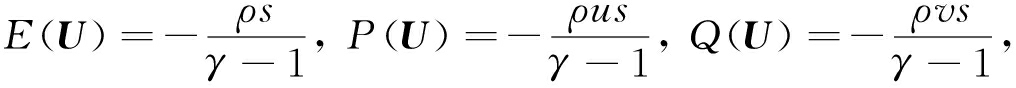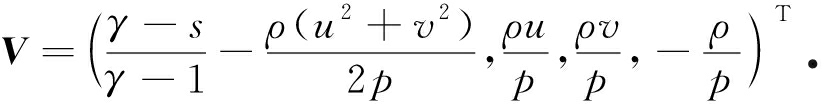### 3.2 HLL通量

HLL格式最早由Harten等提出，该格式是直接求解单元交界面的数值通量，其主要思想是假设精确解的波形图是由两条波分隔成三个常数区域．HLL格式给出了计算单元通量波速的方法.

HLL通量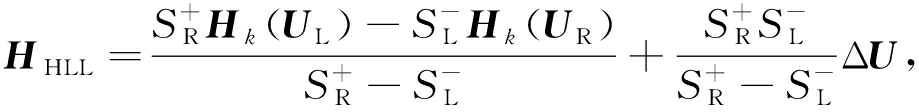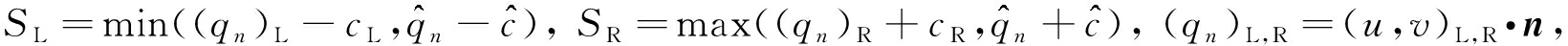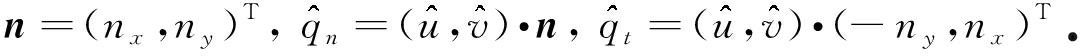Roe均值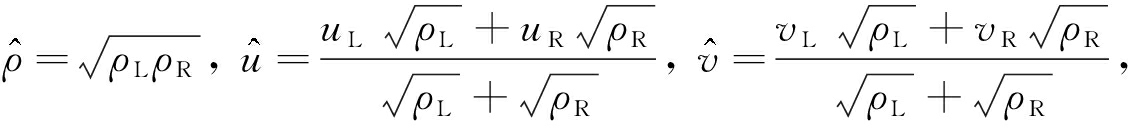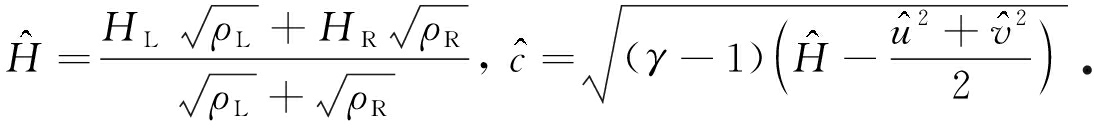### 3.3 混合格式

n=α1n1+α2n2α1=n·n1α2=n·n2

Hm=α1HHLL+α2HES.

## 4 数 值 算 例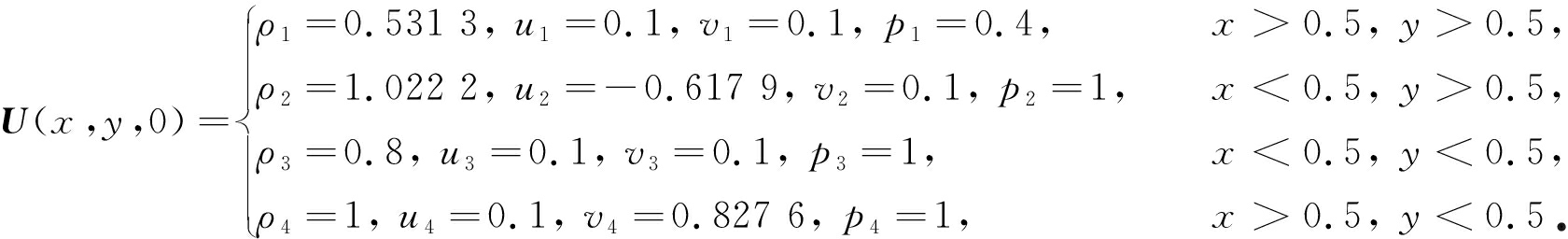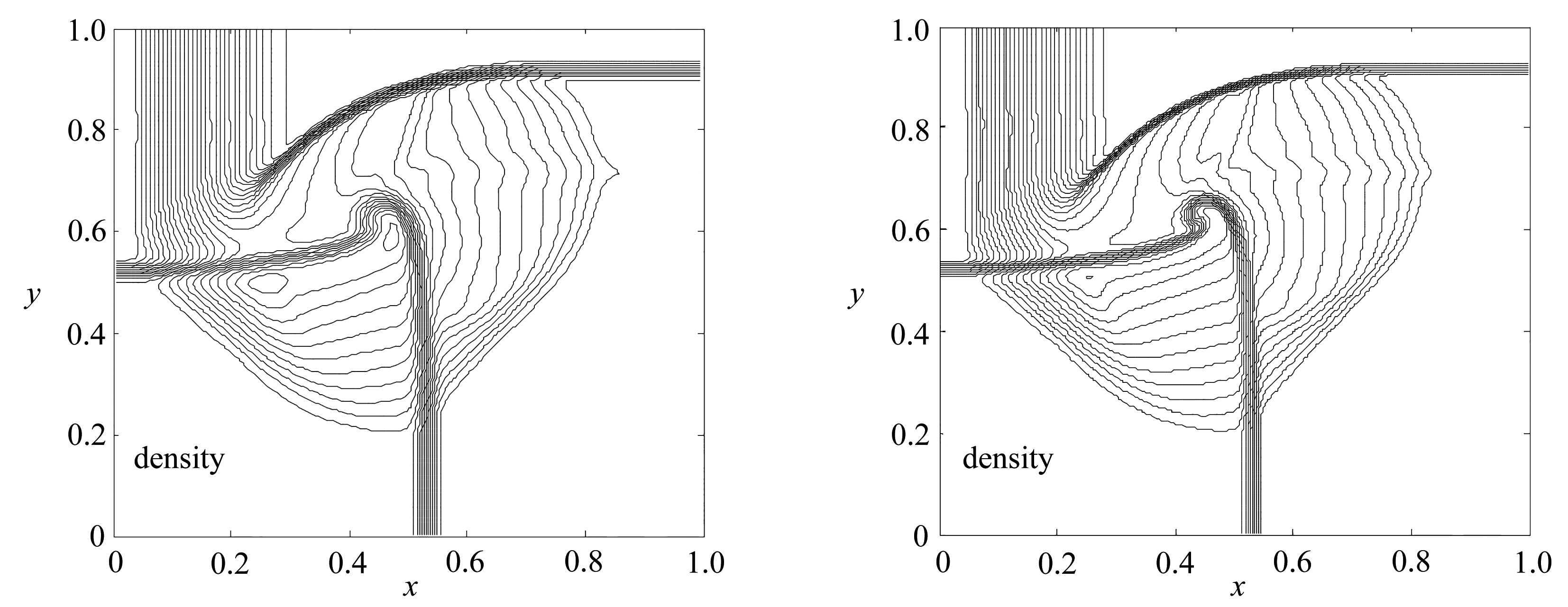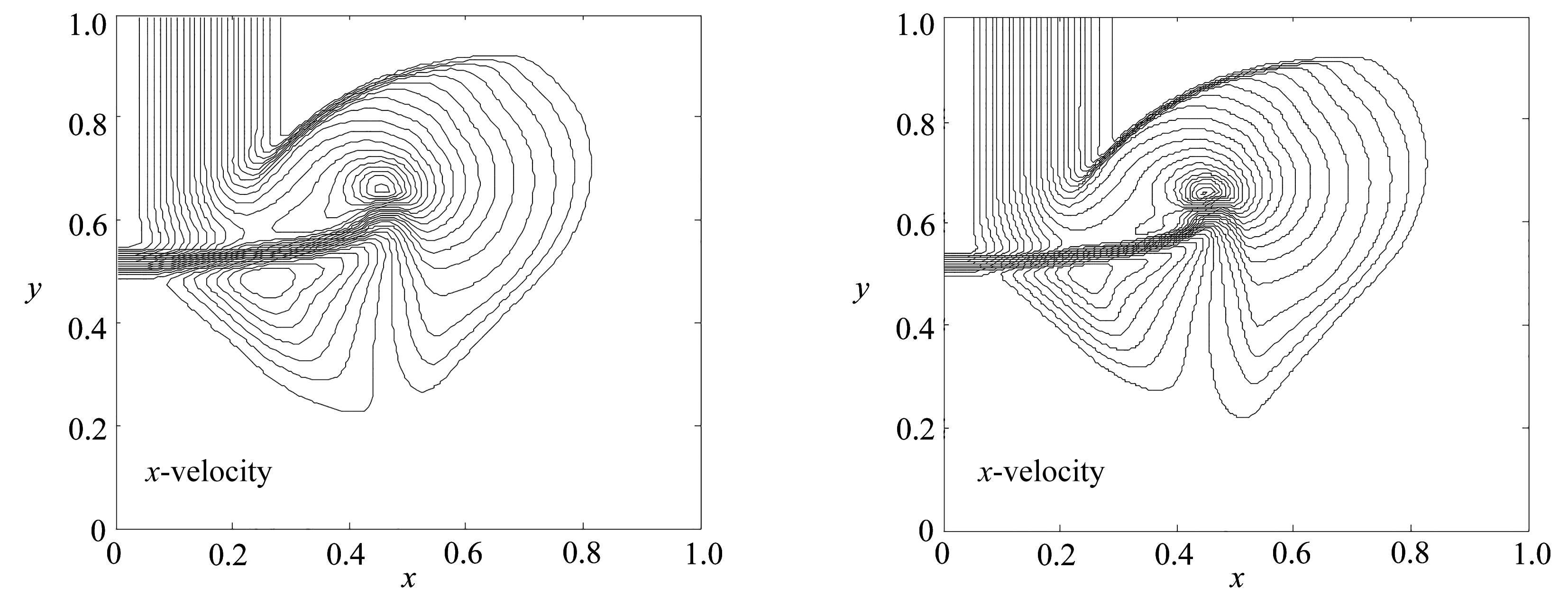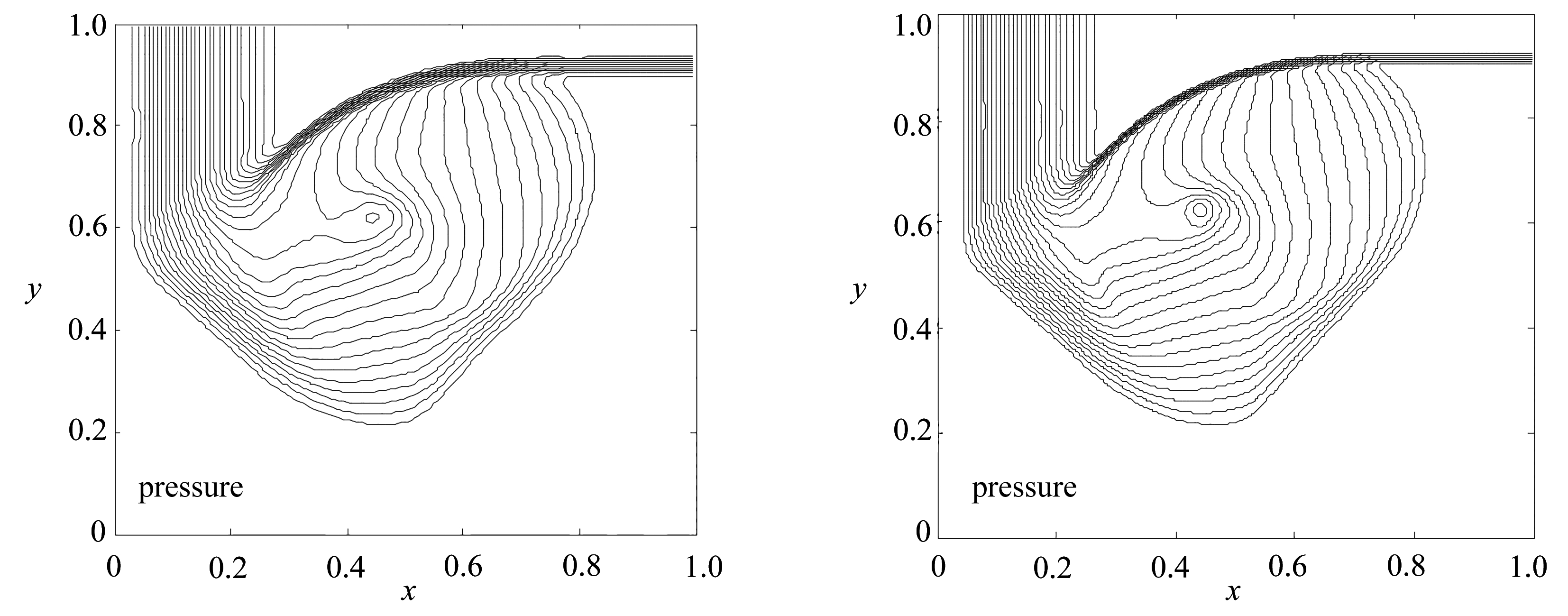(a) 非混合格式结果 (b) 混合格式结果
(a) Non-mixed scheme results (b) Mixed scheme results

Fig. 1 Contours(case 1)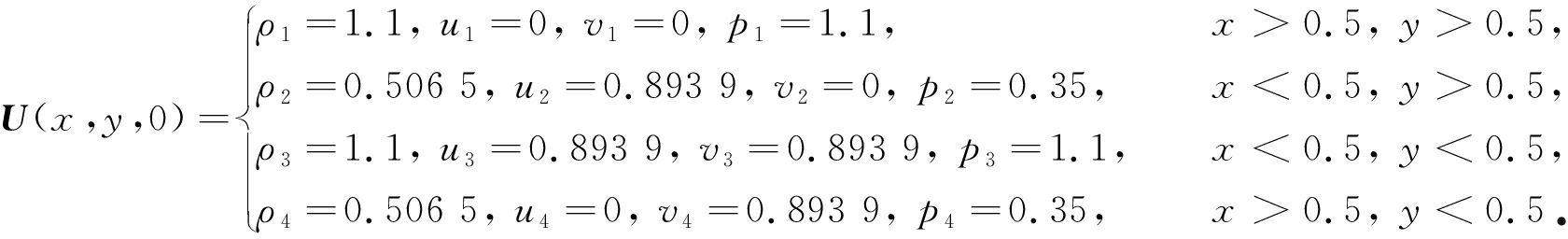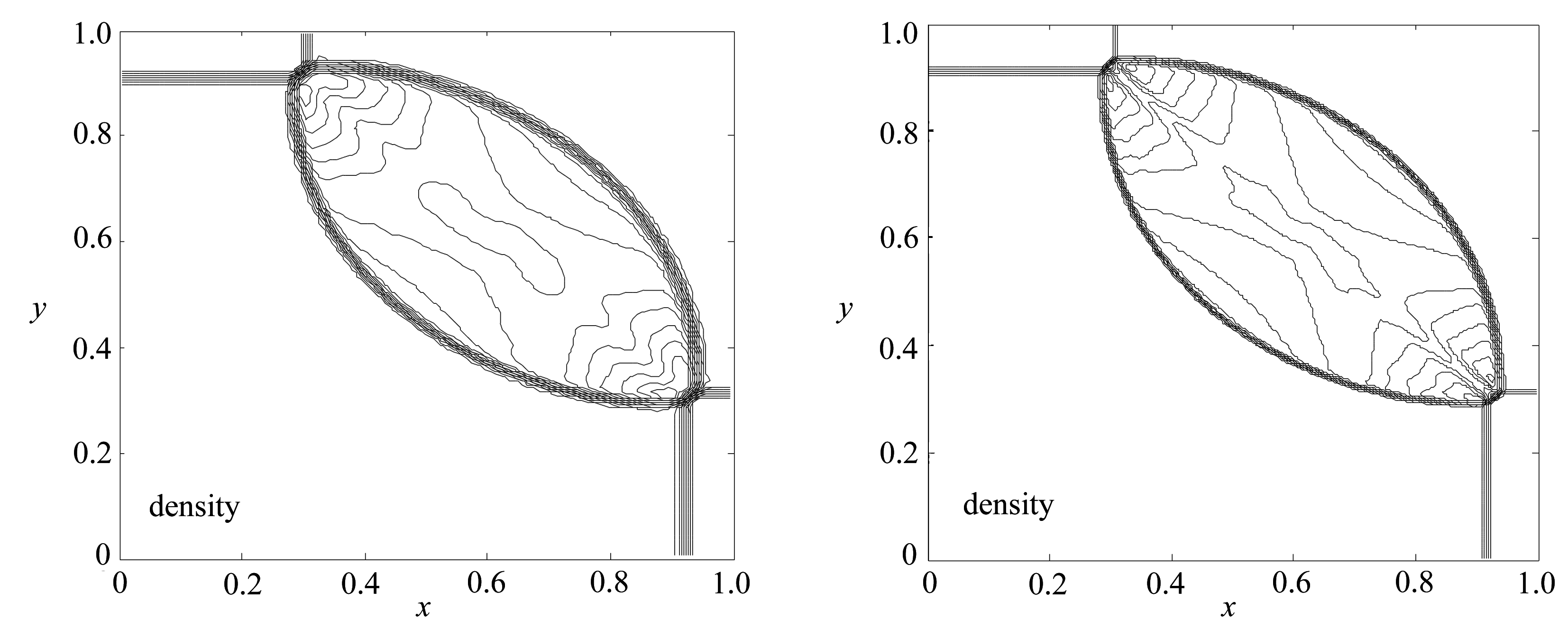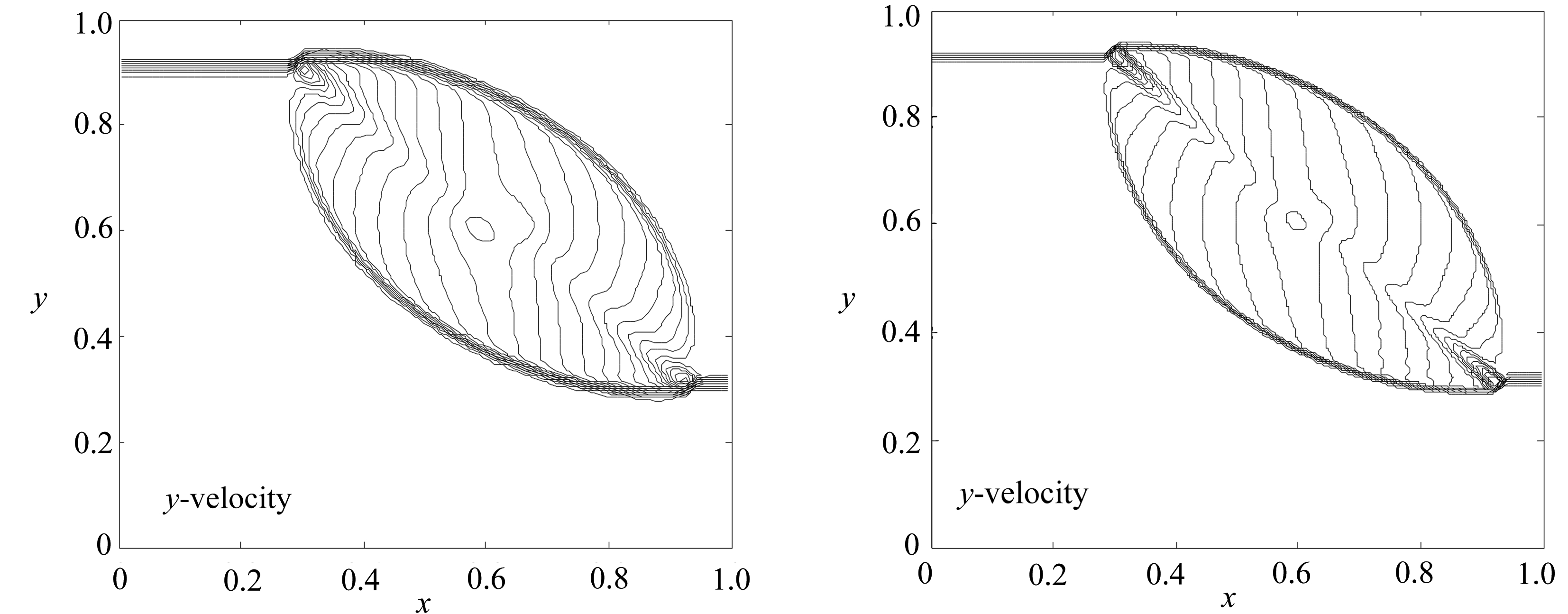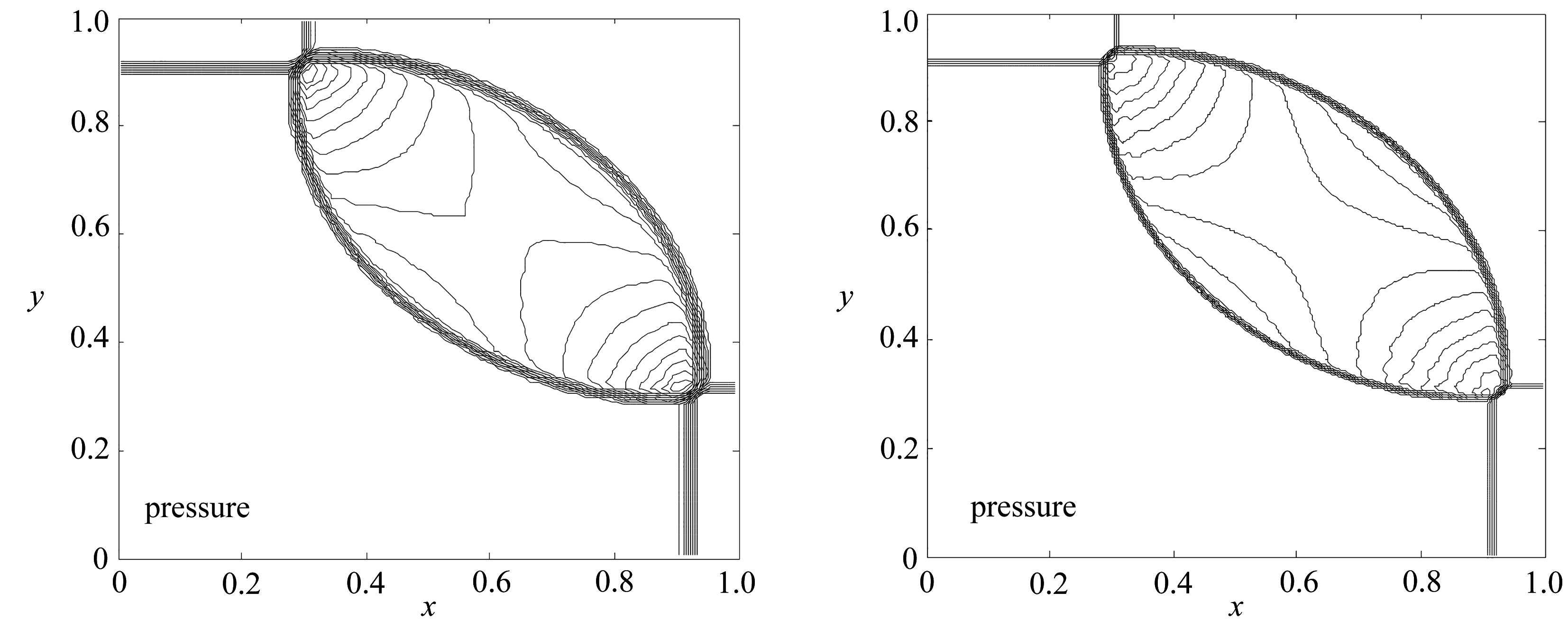(a) 非混合格式结果 (b) 混合格式结果
(a) Non-mixed scheme results (b) Mixed scheme results

Fig. 2 Contours(case 2)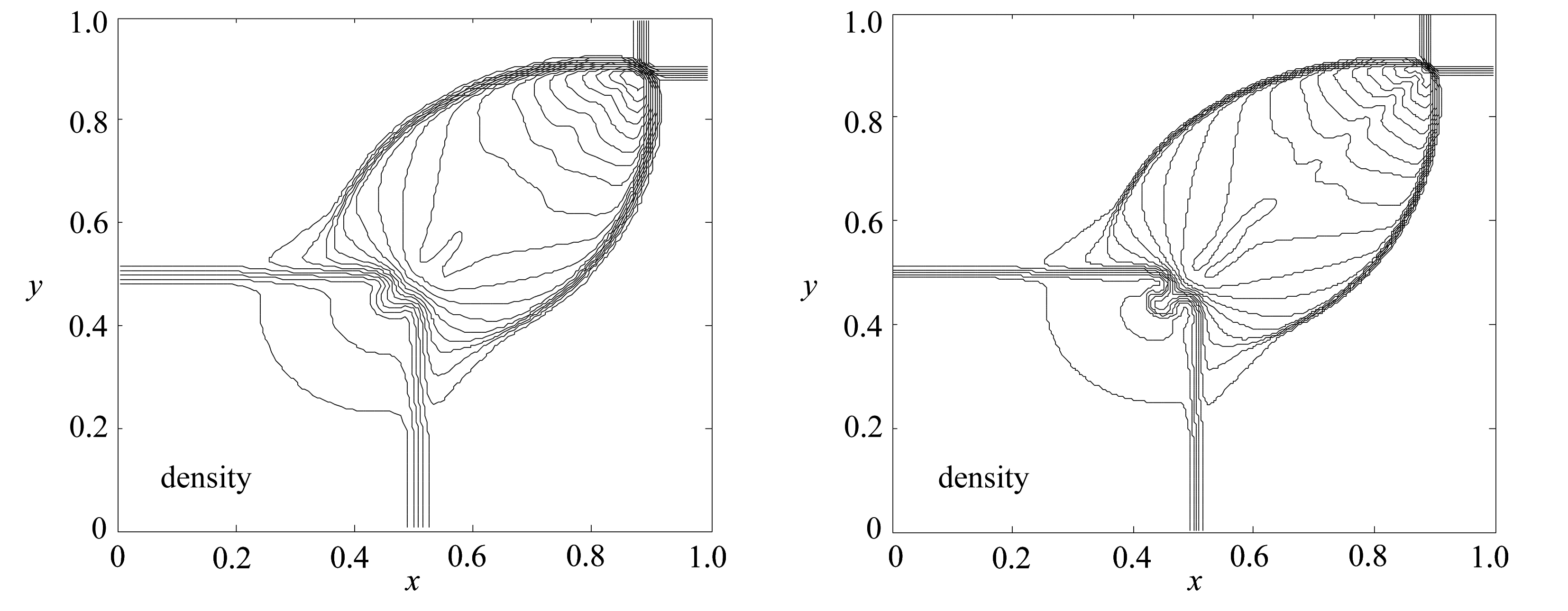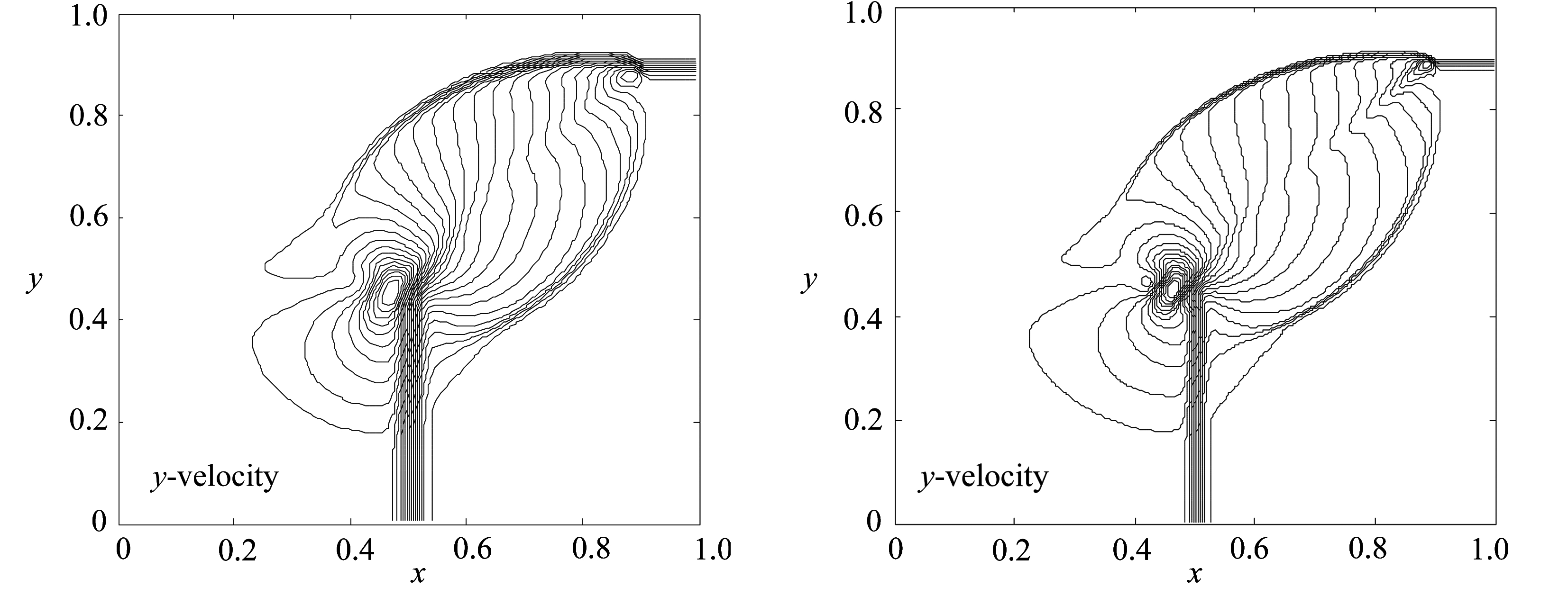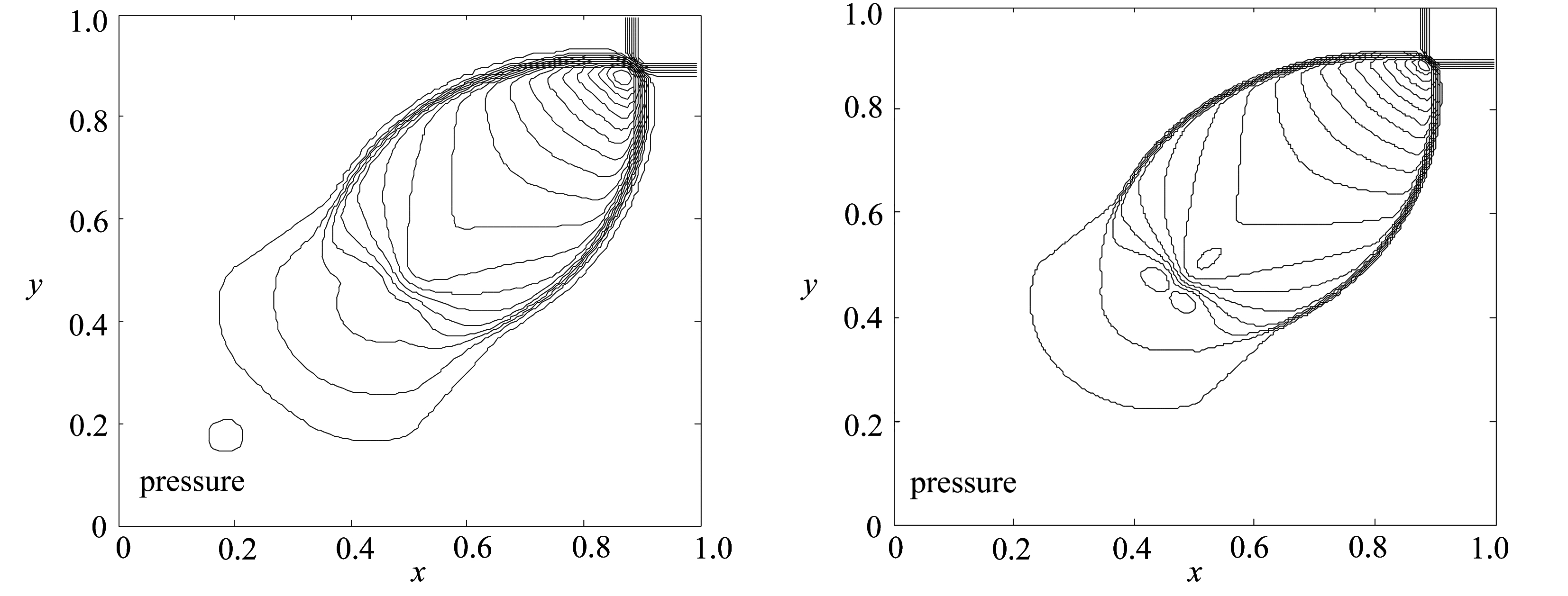(a) 非混合格式结果 (b) 混合格式结果
(a) Non-mixed scheme results (b) Mixed scheme results

Fig. 3 Contours(case 3)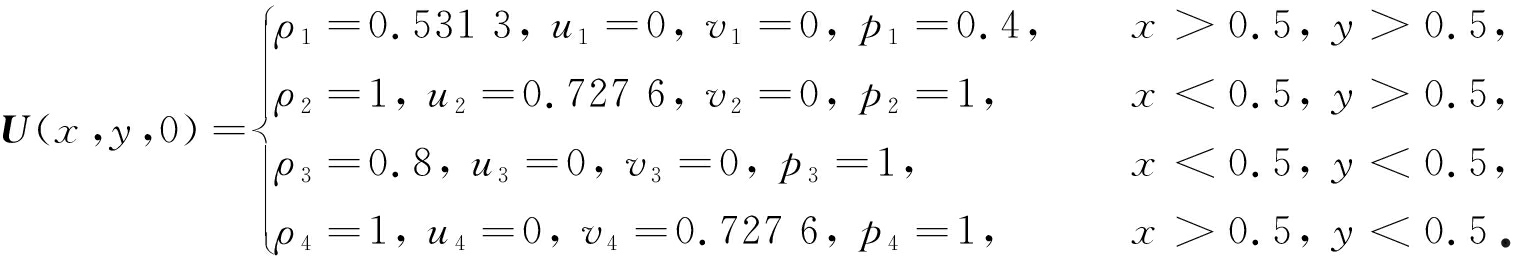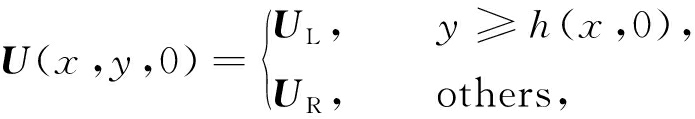UL=[8,57.259 7, -33.001 2, 563.544]T,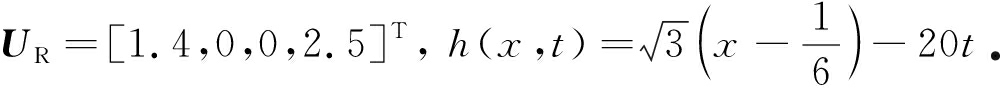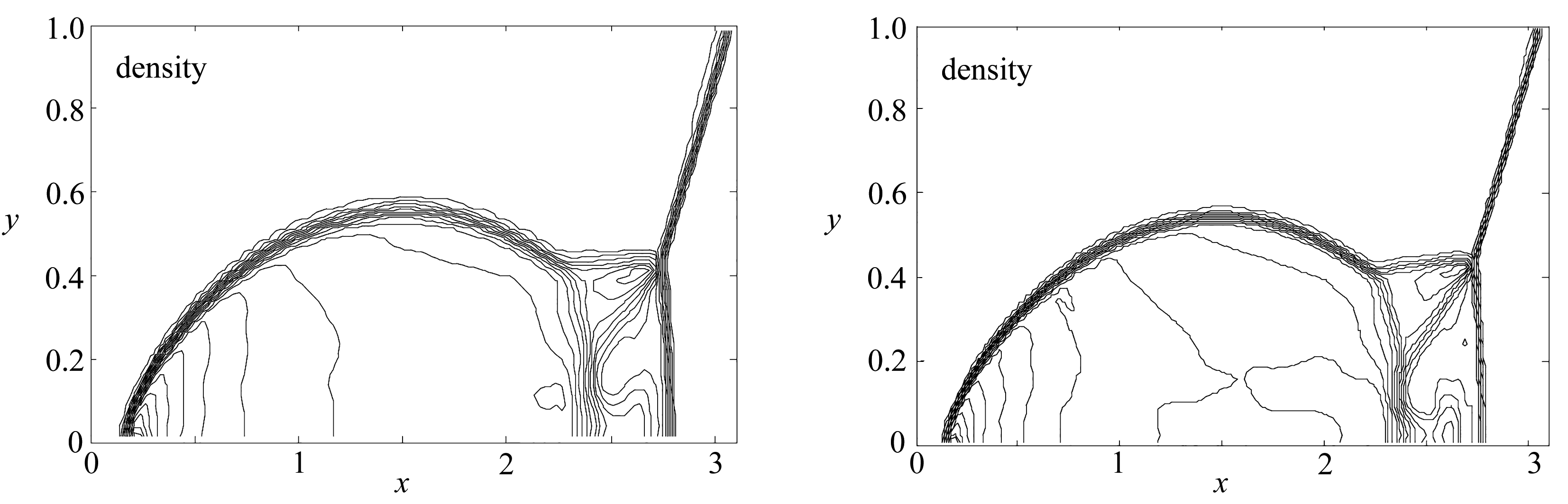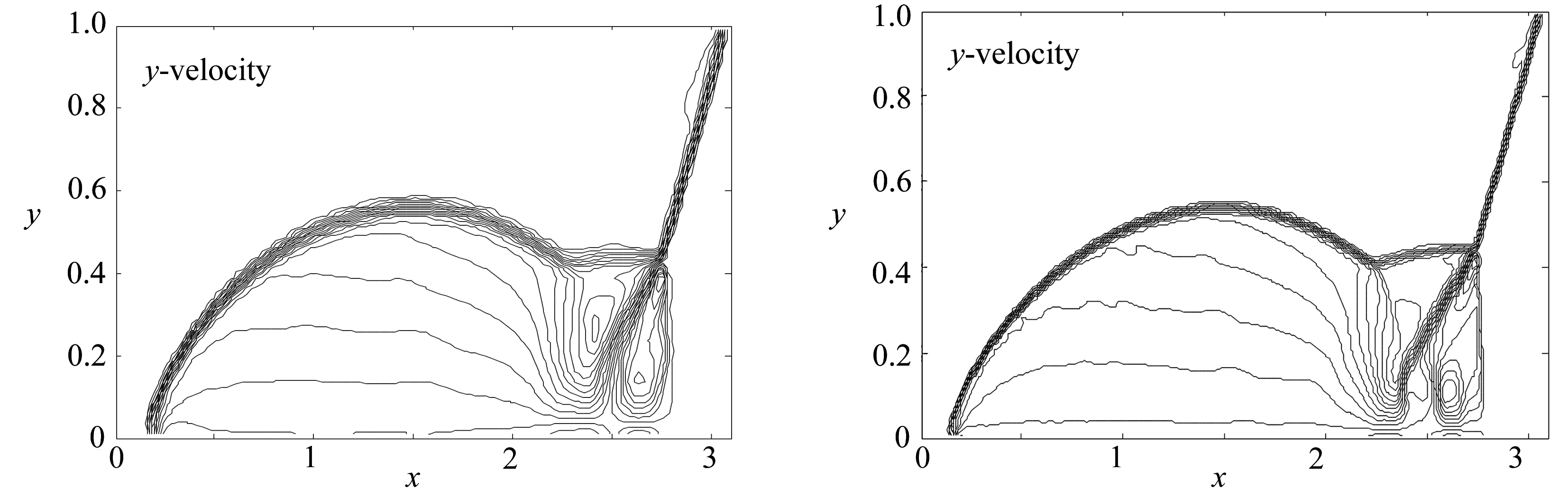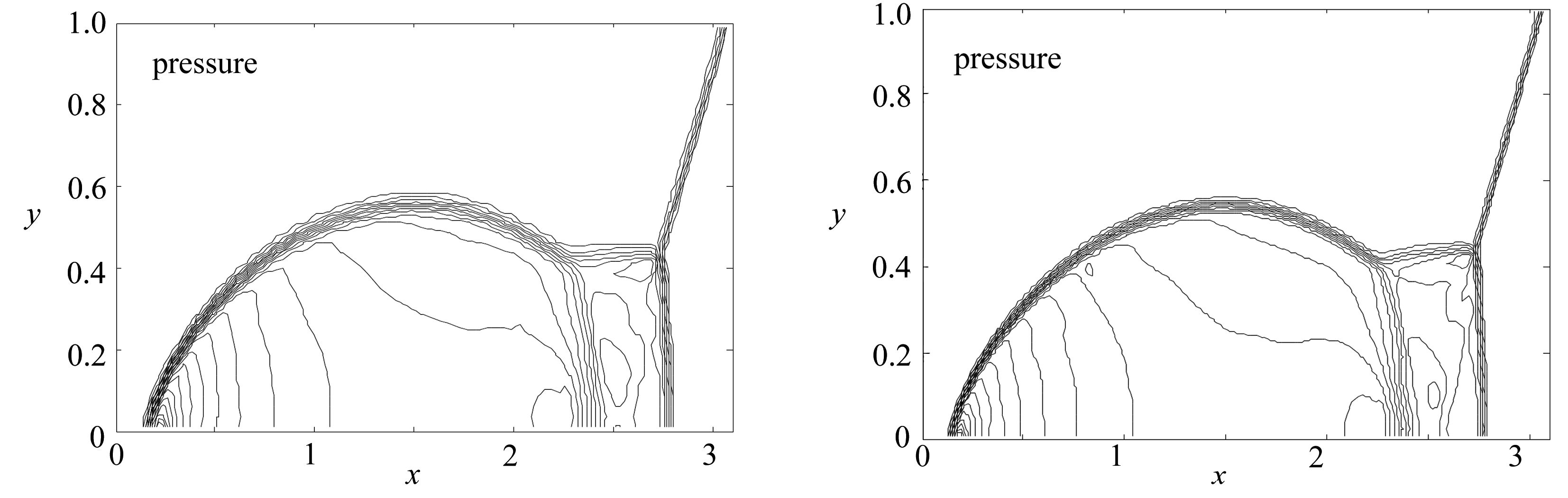(a) 非混合格式结果 (b) 混合格式结果
(a) Non-mixed scheme results (b) Mixed scheme results

Fig. 4 Contours(case 4)

## 5 总 结

 GODUNOV S. Finite difference method for numerical computation of discontinuous solutions of the equations of fluid dynamics[J].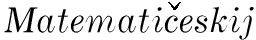Sbornik, 1959, 47(2): 271-306.

 VAN LEER B. Towards the ultimate conservative difference scheme, Ⅴ: a second-order sequel to Godunov’s method[J]. Journal of Computational Physics, 1979, 32(1): 101-136.

 ROE P. Approximate Riemann solvers, parameter vectors, and difference schemes[J]. Journal of Computational Physics, 1981, 43(1): 357-372.

 LION M S. Mass flux schemes and connection to shock instability[J]. Journal of Computational Physics, 2000, 160(2): 623-648.

 PANDOLFI M, AMBROSIO D. Numerical instabilities in upwind methods: analysis and cures for the “carbuncle” phenomenon[J]. Journal of Computational Physics, 2001, 166(2): 271-301.

 QUIRK J J. A contribution to the great Riemann solver debate[J]. International Journal for Numerical Methods in Fluids, 1994, 18(6): 550-569.

 SANDERS R, MORANO E, DRUGUET M. Multidimensional dissipation for upwind schemes: stability and applications to gas dynamics[J]. Journal of Computational Physics, 1998, 145(2): 511-537.

 LEVY D W, POWELL K G, VAN LEER B. Use of a rotated Riemann solver for the two-dimensional Euler equations[J]. Journal of Computational Physics, 1993, 106(2): 201-214.

 REN Y X. A robust shock-capturing scheme based on rotated Riemann solvers[J]. Computer & Fluids, 2003, 32(6): 1379-1403.

 NISHIKAWA H, KITAMURA K. Very simple, carbuncle-free, boundary-layer-resolving, rotated-hybrid Riemann solvers[J]. Journal of Computational Physics, 2008, 227(4): 2560-2581.

 ZHANG F, LIU J. Evaluation of rotated upwind schemes for contact discontinuity and strong shock[J]. Computer & Fluids, 2016, 134: 11-22.

 刘友琼, 封建湖, 任烔, 等. 求解多维Euler方程的二阶旋转混合型格式[J]. 应用数学和力学, 2014, 35(5): 542-553.(LIU Youqiong, FENG Jianhu, REN Tong, et al. Second order rotational hybrid scheme for solving multi-dimensional Euler equation[J]. Applied Mathematics and Mechanics, 2014, 35(5): 542-553.(in Chinese))

 LAX P D. Weak solutions of nonlinear hyperbolic equations and their numerical computation[J]. Communications on Pure and Applied Mathematics, 1954, 7(1): 159-193.

 ISMAIL F, ROE P L. Affordable, entropy-consistent Euler flux functions Ⅱ: entropy production at shocks[J]. Journal of Computational Physics, 2009, 228(4): 5410-5436.

 TADMOR E. Entropy stable approximations of Navier-Stokes equations with no artificial numerical viscosity[J]. Journal of Hyperbolic Differential Equations, 2006, 3(3): 529-559.

 郑素佩, 王令, 王苗苗. 求解二维浅水波方程的移动网格旋转通量法[J]. 应用数学和力学, 2020, 41(1): 42-53.(ZHENG Supei, WANG Ling, WANG Miaomiao. Solution of 2D shallow water wave equation with the moving-grid rotating-invariance method[J]. Applied Mathematics and Mechanics, 2020, 41(1): 42-53.(in Chinese))

 TORO F. Riemann Solvers and Numerical Methods for Fluid Dynamics[M]. Berlin: Springer, 2013.

 SHU C W. Essentially non-oscillatory and weighted essentially non-oscillatory schemes for hyperbolic conservation laws[J]. National Aeronautics and Space Administration, 1997, 97(1): 206-253.

 TADMOR E. The numerical viscosity of entropy stable schemes for systems of conservation laws: Ⅰ[J]. Mathematics of Computation, 1987, 49(179): 91-103.

 TADMOR E. Entropy stability theory for difference approximations of nonlinear conservation laws and related time-dependent problems[J]. Acta Numerica, 2003, 12: 451-512.

 HARTEN A, LAX P D, VAN LEER B. On upstream differencing and Godunov-type schemes for hyperbolic conservation laws[J]. SIAM Review, 1983, 25(1): 35-61.

 LAX P, LIU X D. Solution of two-dimensional Riemann problems of gas dynamics by positive schemes[J]. SIAM Journal on Scientific Computing, 1998, 19(2): 319-340.

 WOODWARD P, COLELLA P. The numerical simulation of two-dimensional fluid flow with strong shocks[J]. Journal of Computation Physics, 1984, 54(1): 115-173.

# A Hybrid Scheme of Rotational Flux for Solving 2D Euler Equations

JIA Dou, ZHENG Supei

(School of Sciences, Changan University, Xian 710064, P.R.China)

Abstract: To improve the resolution of the numerical results for the 2D Euler equations, a hybrid scheme of rotational flux was proposed. The algorithm was built under the quasi-1D idea of the rotational flux method, and the flux function was given in the form of a hybrid scheme coupling the entropy stable numerical flux satisfying the 2nd law of thermodynamics with the HLL numerical flux of good robustness. The time was advanced with the 3rd-order strongly stable Runge-Kutta method. The hybrid scheme of rotational flux has the advantages of simple structure and high resolution. Numerical results show good characteristics of the algorithm.

Key words: rotational invariance; entropy stable scheme; HLL scheme; Euler equation

DOI：10.21656/1000-0887.410196

*收稿日期： 2020-06-30； 修订日期：2020-07-19